This blog is managed by Song Hock Chye, author of Improve Your Thinking Skills in Maths (P1-P3 series), which is published and distributed by EPH.

## Monday, April 28, 2008

### Ai Tong School P5 SA1 2006 Math (Q47)

Some villagers were building a bridge across a river. On the first week, they built 210 m of the bridge. On the second week, they built 1/5 of the remaining length. How long was the bridge if the total length that they had built on the first two weeks was twice the remaining length to be built?

Solution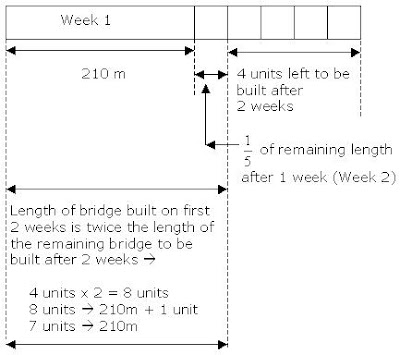7 units ----- 210
1 unit ----- 210 divided by 7 = 30

Length of bridge ----- 210m + 5 units
= 210m + (5 x 30m)
= 210m + 150m
= 360m

Answer: The length of the bridge is 360m.

## Friday, April 25, 2008

### Ai Tong School P5 SA1 2006 Math (Q45)

At Ai Tong Restaurant, the charge for the buffet lunch per person is \$15. For every group of 10 people, the 11th person does not have to pay.

(a) What will be the total charge for a party of 23 people?
(b) How many people are there in the group if the bill comes up to \$720?

Solution

10 people ----- 10 x \$15 = \$150
11th person ----- free
1 group of 11 ----- \$150

(a) What will be the total charge for a party of 23 people?

23 people ----- 2 groups of 11 people + 1 individual
2 groups of 11 ----- 2 x \$150 = \$300
1 individual ----- \$15
Total ----- \$300 + \$15 = \$315

Answer: The charge for a party of 23 people is \$315.

(b) How many people are there in the group if the bill comes up to \$720?

For every \$150 ----- 11 people
\$720 ----- \$600 + \$120
\$600 ----- \$600 divided by \$150 = 4 (groups of 11 people)
\$120 ----- \$120 divided by \$15 = 8 individuals

Therefore, \$720 ----- 4 groups of 11 people + 11 individuals
(4 x 11) + 8
= 44 + 8
= 52

Answer: There are 52 people if the bill comes up to \$720.

## Wednesday, April 23, 2008

### Ai Tong School P5 SA1 2006 Math (Q44)

ABCD is a square. AD = 48 cm. Given that DN is twice as long as NC and BM = MC, find the area of the unshaded part.

SolutionArea of Triangle ADN ----- ½ x base x height
= ½ x 32 cm x 48 cm = 768 square cm

Area of OMCN ----- 24 cm x 16 cm = 384 square cm

Total unshaded area ----- (768 + 384) square cm = 1152 square cm (Answer)

## Monday, April 21, 2008

### Nanyang Primary School P5 SA2 2006

Posted by Anonymous 21 Apr 2008 PM 01:48
Nanyang Primary SchoolSA2 2006 Primary 5

Three girls shared a sum of money. Cathy received \$180 more than Betty. The total amount Betty and Cathy received was 3 times the amount Alice received. The amount Alice and Cathy received was 5 times the amount Betty received. What was the sum of money shared by the three girls?

pls enlighten. thanks

(As time does not permit, please note that this will be my last post in response to requests from the public. I will however still post worked solutions from my own database and requests from my own students)Cathy received \$180 (3 groups of \$60) more than Betty.

Betty + Cathy ----- 6 units + 3 groups of \$60

Alicia has one third of that -----
(6 units)/3 + (3 groups of \$60)/3
= 2 units + \$60

At the same time, Alice and Cathy received 5 times the amount Betty received. Therefore, Betty’s amount has to be multiplied 5 times to be equal to the total of amount of Alice and Cathy.

Betty x 5 ----- Alicia + Cathy
(3 units x 5) ----- (2 units + \$60) + (3 units + \$180)
15 units ----- 5 units + \$240
10 units ----- \$240
1 unit ----- \$24

Total sum of money -----
8 units + \$240
= (8 x \$24) + \$240
= \$192 + \$240 = \$432

## Sunday, April 20, 2008

### Common Science Mistake – Internal and External Fertilisation

Fertilisation (in animals) is the process where the male sperm cell fuses with the female egg cell.

Internal fertilisation occurs when the male sperm cell fuses with the female egg cell, in the female’s body.

External fertilisation occurs when the male sperm cell fuses with the female egg cell, outside the female’s body.

The most common mistake made by students is that they believe internal fertilisation occurs in animals that give birth (example mammals) and external fertilisation occurs in animals that lay eggs (example birds and reptiles etc). This concept is wrong.

Internal fertilisation occurs in mammals, birds and reptiles.

In the case of mammals, fertilisation occurs in the female’s body. The new life develops inside the mother’s womb for most mammals.

In the case of birds and reptiles, fertilisation occurs inside the female’s body, and the fertilised egg is then laid. The new life develops outside the mother’s body.

In the case for amphibians and most fishes, fertilisation occurs outside the female’s body and the new life develops outside the mother’s body.

Below are some examples of internal and external fertilisation.## Thursday, April 17, 2008

### Ai Tong School P5 SA1 2006 Math (Q43)

The cost of a kiwi fruit was ¼ that of a rock melon. Melvin spent 4/7 of his money on 12 rock melons and 4 kiwi fruits. Then he used the remaining amount of money to buy 3 more rock melons and some kiwi fruits. How many kiwi fruits did he buy in all?

Solution

Kiwi fruit ----- 1 unit
Rock melon ----- 4 units

12 rock melons + 4 kiwi fruits ----- 4/7 of money
(12 x 4 units) + (4 units) ----- 4/7 of money
48 units + 4 units ----- 4/7 of money
52 units ----- 4/7 of money

4/7 of money ----- 52 units
1/7 of money ----- 52 units divided by 4 = 13 units

The remaining amount of money he had was 3/7, therefore
3/7 of money ----- 13 units x 3 = 39 units

He used this amount of money to buy 3 rock melons and some kiwi fruits.

3 rock melons + unknown no. of kiwi fruits ----- 39 units
(3 x 4 units) + ? ----- 39 units
12 units + ? ----- 39 units
? ----- 39 units – 13 units = 27 units

1 unit ----- 1 kiwi fruit
27 units ----- 27 kiwi fruits

Total no. of kiwi fruits bought -----
4/7 of money was spent on 12 rock lemons and 4 kiwi fruits.
3/7 of money was spent on 3 rock lemons and 27 kiwi fruits.
Total no. of kiwi fruits ----- 4 + 27 = 31

Answer: He bought a total of 31 kiwi fruits.

## Tuesday, April 15, 2008

### More than one way to solve Math problem sums

Anonymous posted on 14 Apr 2008 PM 10:12

isn't it faster to do this:
Ratio 20 cents: 50 cents : \$1
= 2 : 4 : 7
2 x 20 cents + 4 x 50 cents + 7X 100 cents = 940 cents
4700 / 940 = 5 groups
5 x (2 + 4 + 7) = 65 coins

The question and solution that I have worked out, that is referred to, can be found in this link.

Question - The ratio of the number of 20-cent coins to the number of 50-cent coins to the number of \$1 coins in a bag is 2 : 4 : 7. Given that the total amount of money in the bag is \$47, how many coins are there altogether?

Dear Anonymous,

There is more than one way to solve Math problem sums. My bottom line, as a teacher when I was teaching in schools, and as a tutor now, is that the method used/taught must contain these important elements –

Presentating the solution in a way such that the student is able to understand its mechanics
This is important. While above average students are able to analyse deeper, average and below average students usually are not able to. As such, the more complex the problem sum, the more there is a need to break down the solution with a step-by-step method.

Transfer of the skill/knowledge used to solve the sum to students
This is the acid test of your teaching method. If a teacher/tutor is unable to transfer his skill and/or knowledge to the student, such that the student is unable to solve the problem sum, should he come across it again in the exam, all effort is wasted.

The complexity of the math question above, requires the ability on the part of the student to differentiate 2 distinct differences. They are:

1. The number of coins.
2. The value of coins.

Your “short” method assumes the student is able to differentiate the above 2 mentally. This can be seen when you wrote:

2 x 20 cents + 4 x 50 cents + 7X 100 cents = 940 cents
4700 / 940 = 5 groups
5 x (2 + 4 + 7) = 65 coins

However, not all students are able to see that there are 5 groups of 940 cents in 4700 cents, let alone the need to multiply the number of coins by its value before you can come to that.

Your last line also requires the student to understand that within one group of the 3 coins of different values in the (2 + 4 + 7), there are 940 cents – which essentially means 5 groups of the same 3 coins of different values (2 + 4 + 7), will contain 65 coins, because the 5 groups are valued at 4700 cents.

Your method is good for above average students.

However, not all students are able to see what you see.

The biggest challenge some students face with a problem sum like the above is, the failure to realize that the number of coins and the value of coins are 2 different things - or – if they do realize they are 2 different things, they do not know how to proceed to solve the sum.

Hence, I would recommend the longer method as I have worked out for the average and below average student. The longer method I have worked out uses the step-by-step approach I mentioned earlier. The steps are –

Step 1 - Converting the number of coins of different denominations into a single common base, which is to consider the values of all the 3 different coins. This is the key to solve the problem because we are given the total value of the coins. This can be seen as shown below.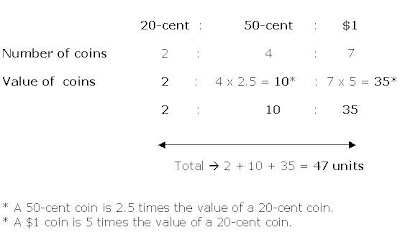Hence, from a ratio of 2 : 4 : 7 of different values, we convert them into a ratio of 2 : 10 : 35, which has a common base (which is the value). Since this ratio concerns value of the coins of each denomination, it can then be compared to the total value of the coins, which is \$47. A common base between the ratio and total value has been established.

Step 2 – The next step easy. By comparing the converted ratio (which is based on value) to the total value of the coins, we can establish that critical “1 unit” as shown below.

47 units ----- \$47 (Total amount of money in bag is \$47)
1 unit ----- \$47 divided by 47 = \$1

Step 3 – The rest of the solution is even simpler to understand and it is self-explanatory.
Value of 20-cent coins ----- 2 units ----- \$2
Value of 50-cent coins ----- 10 units ----- \$10
Value of \$1 coins ----- 35 units ----- \$35

No. of 20-cent coins ----- \$2 divided by 20 cents ----- 10 coins
No. of 50-cent coins ----- \$10 divided by 50 cents ----- 20 coins
No. of \$1 coins ----- \$35 divided by \$1 ----- 35 coins

Total number of coins in the bag, 10 + 20 + 35 = 65
Answer: There were 65 coins in the bag.

Conclusion -
There is nothing wrong with your “short method”. My only concern is that weaker students may be unable to see as deeply as you are able to. I have noticed that many tuition centres teach “fast methods”.

However, it must be remembered that these “fast methods” are effective if the students are bright. The 2 essential points I mentioned earlier, which are, presenting the solution in a manner such the student understands its mechanics, and transferring that skill to solve the sum to the student such that he will be able to solve the same problem sum should he come across it in exams, may be compromised, if the student is weak in maths.

Average students and below average students need a step-by-step approach.

The purpose of this blog is to help average and below average students. As such, you may notice that most of the solutions follow a step-by-step approach.

## Monday, April 14, 2008

### Ai Tong School P5 SA1 2006 Math (Q42)

800 people attended the 2006 New Year’s party. 2/5 of the men and ½ of the women are Malay adults. There were an equal number of male and female Chinese adults. There were also 140 children at the party.

(a) How many female Chinese adults were at the party?
(b) What fraction of the people attending the party were male Malay adults?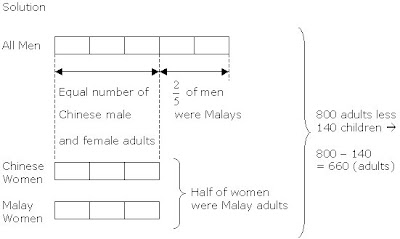11 units ----- 660
1 unit ----- 660 divided by 11 = 60

(a) How many female Chinese adults were at the party?

(Chinese women) 3 units ----- 60 x 3 = 180

Answer: There were 180 Chinese women

(b) What fraction of the people attending the party were male Malay adults?

(Malay men) 2 units ----- 60 x 2 = 120

Fraction of people who were male Malay adults -----

120/800 = 3/20

Answer: The fraction of people who were male Malay adults was 3/20.

## Thursday, April 10, 2008

### Ai Tong School P5 SA1 2006 Math (Q41)

Jenny paid \$364 for 6 dresses and 2 belts. Each dress cost 4 times as much as a belt. Find the total cost of 3 belts.

Solution24 units + 2 units ----- \$364
26 units ----- \$364
1 unit ----- \$364 divided by 26 = \$14

(3 belts) 3 units ----- \$14 x 3 = \$42

### Reply to Alex from Cbox

Question posted by Alex as seen below….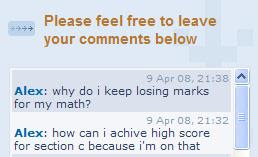You did not mention what is your score for Sect C or the other 2 sections. If you can score around 38 marks and above for your combined Sect A/B, but less than 25 for Sect C, it means that you lack practice. You should start practising Section C type questions.

However, if you score below 25 marks for Sect A and B combined, you need more than just practice. In all probability, your foundation topics may be weak. If that is the case, you need to strengthen your foundation by doing topical test papers.

In any case, the best person to consult is your Maths teacher. She will be able to identify your weak areas. A teacher is paid to teach. So there is no need to be afraid to ask her.

## Monday, April 07, 2008

### Ai Tong School P5 SA1 2006 Math (Q40)

Pei Chai and Jia Liang shared a box of cards in the ratio of 4 : 1. After Pei Chai gave Jia Liang 45 of his cards, they had the same number of cards. How many cards did Jia Liang have at first?

Solution3 units ----- 45
1 unit ----- 45 divided by 3 = 15
(Jia Liang at first) 2 units ----- 15 x 2 = 30

## Friday, April 04, 2008

### Ai Tong School P5 SA1 2006 Math (Q39)

ABCD is a rectangle (not drawn to scale). AX = XD and AY = YB. Find the area of triangle CXY.

Solution

Area of rectangle ----- length x breadth
= 14 cm x 8 cm = 112 square cm

Area of Triangle CDX ----- ½ x base x height
= ½ x 14 cm x 4 cm = 28 square cm

Area of Triangle AXY ----- ½ x 7 cm x 4 cm = 14 square cm

Area of Triangle CBY ----- ½ x 7 cm x 8 cm = 28 square cm

Area of Triangle CXY -----
Area of rectangle – Area of Triangle CDX – Area of Triangle AXY – Area of Triangle BCY
= (112 – 28 – 14 – 28) square cm
= 42 square cm

Answer: Area of Triangle CXY is 42 square cm.

## Wednesday, April 02, 2008

### Ai Tong School P5 SA1 2006 Math (Q38)

Amy kept 3/5 of her salary and gave the rest to her parents. After spending \$800 on a handphone, she deposited 1/5 of the remaining amount. How much did she deposit if her parents received \$2250?

Solution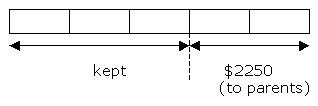2 units ----- \$2250
1 unit ----- \$2250 divided by 2 = \$1125

Amy kept 3 units ----- \$1125 x 3 = \$3375
Spent of phone ----- \$800
Balance ----- \$3375 - \$800 = \$2575

Deposited 1/5 of balance ----- 1/5 x \$2575 = \$515